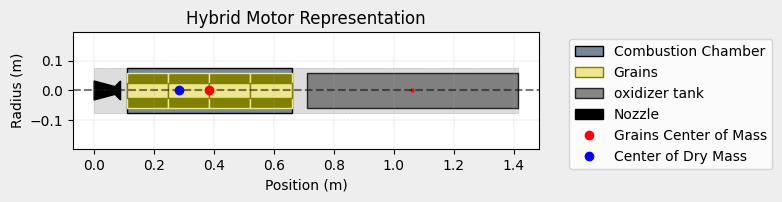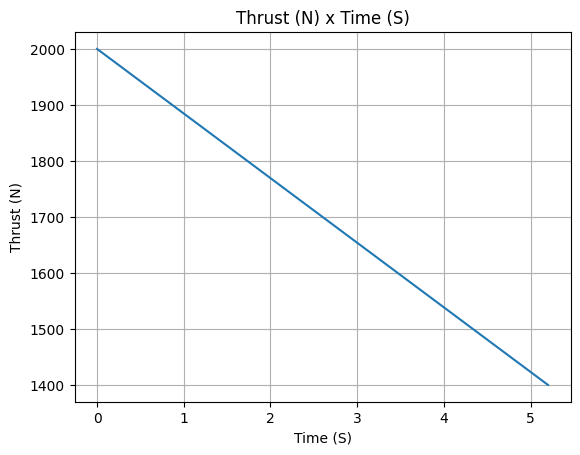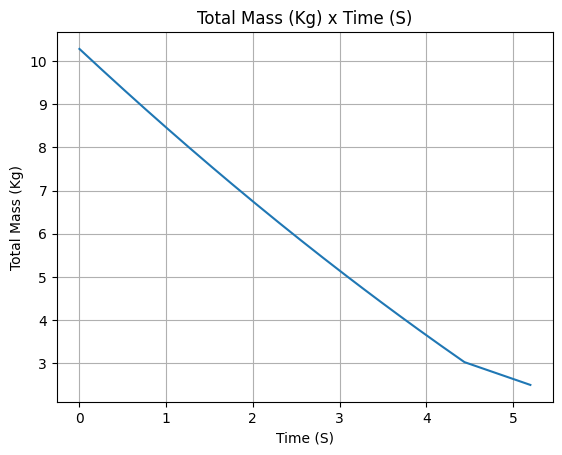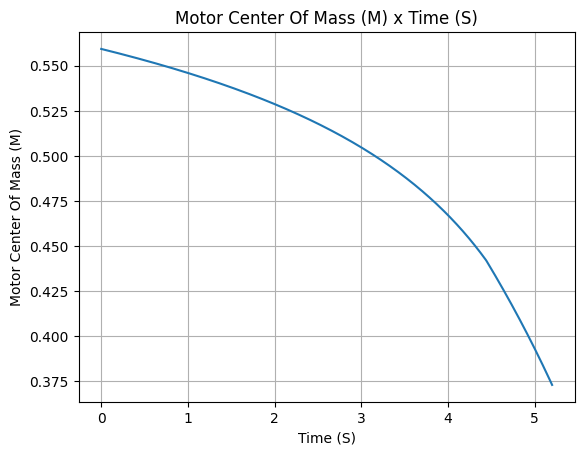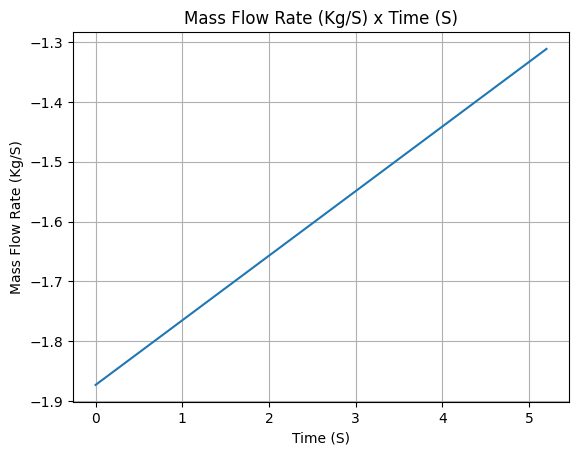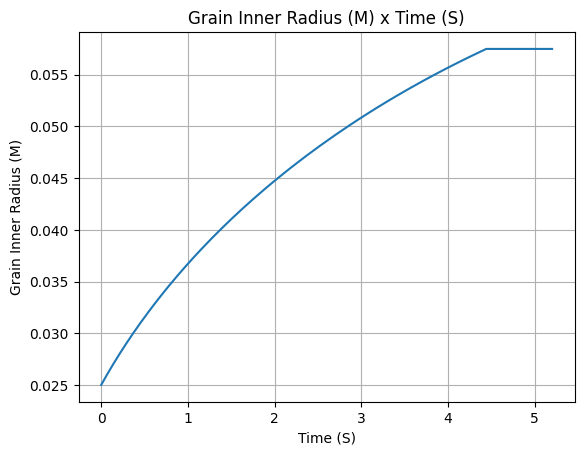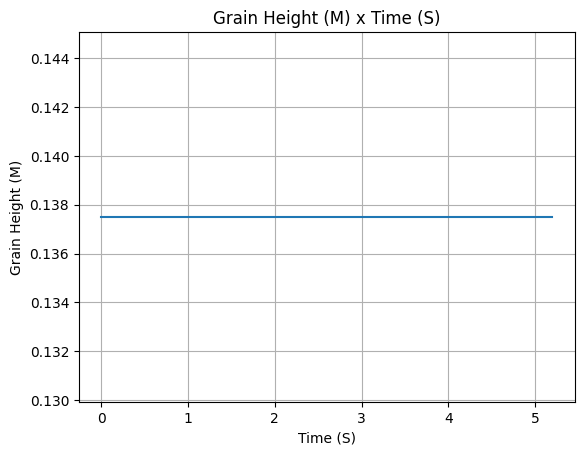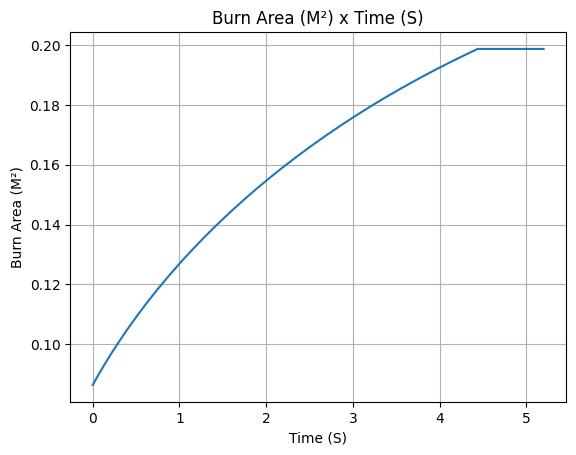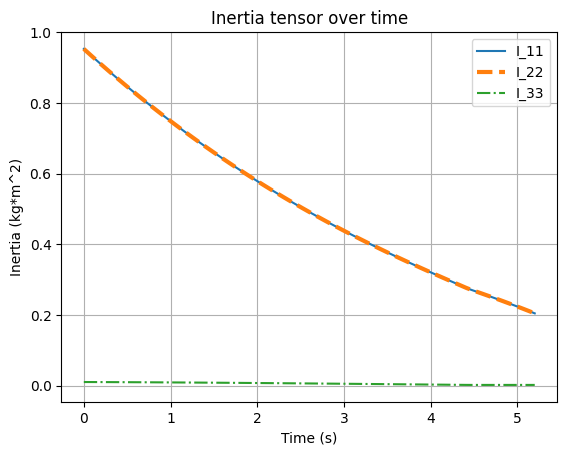# HybridMotor Class Usage#

Here we explore different features of the HybridMotor class.

A hybrid motor is motor composed by a solid fuel grain and a liquid oxidizer. The solid fuel grain is usually made of a rubber-like material, and the liquid oxidizer is usually nitrous oxide. The solid fuel grain is ignited by a spark plug, and the liquid oxidizer is injected into the combustion chamber through an injector. The combustion of the solid fuel grain and the liquid oxidizer generates the thrust.

When simulating this kind of motor in RocketPy, we internally use two motors: one for the solid fuel grain and another for the liquid oxidizer.

The solid fuel behavior is simulated by a solid motor, and the liquid oxidizer behavior is simulated by a liquid motor. The combination of these two create a valid hybrid motor. Everything is then defined at the same time using the HybridMotor class.

## Creating a Hybrid Motor#

To define a hybrid motor, we will need a few information about our motor:

• The thrust source file, which is a file containing the thrust curve of the motor. This file can be a .eng file, a .rse file, or a .csv file. See more details in Thrust Source Details

• A Tank object, which defines the liquid oxidizer tank. See more details in Tank Usage

• Solid fuel parameters, such as the number of grains, their geometry, and their density

• Position related parameters, such as the position of the center of mass of the dry mass, the position of the center of mass of the grains, and the position of the nozzle. See more details in Motor Coordinate Systems

So, lets first import the necessary classes:

```from rocketpy import Fluid, CylindricalTank, MassFlowRateBasedTank, HybridMotor
```

Then we must first define the oxidizer tank:

Tank Usage

```# Define the fluids
oxidizer_liq = Fluid(name="N2O_l", density=1220)
oxidizer_gas = Fluid(name="N2O_g", density=1.9277)

# Define tank geometry
tank_shape = CylindricalTank(115 / 2000, 0.705)

# Define tank
oxidizer_tank = MassFlowRateBasedTank(
name="oxidizer tank",
geometry=tank_shape,
flux_time=5.2,
initial_liquid_mass=4.11,
initial_gas_mass=0,
liquid_mass_flow_rate_in=0,
liquid_mass_flow_rate_out=(4.11 - 0.5) / 5.2,
gas_mass_flow_rate_in=0,
gas_mass_flow_rate_out=0,
liquid=oxidizer_liq,
gas=oxidizer_gas,
)
```

Note

This tank is a MassFlowRateBasedTank, which means that the mass flow rates of the liquid and gas are defined by the user. In this case, the tank has only a liquid mass flow rate out, which is constant.

Now we can define our hybrid motor. We are using a lambda function as the thrust curve, but keep in mind that you can use different formats here.

```example_hybrid = HybridMotor(
thrust_source=lambda t: 2000 - (2000 - 1400) / 5.2 * t,
dry_mass=2,
dry_inertia=(0.125, 0.125, 0.002),
grain_number=4,
grain_separation=0,
grain_initial_height=0.1375,
grain_density=900,
grains_center_of_mass_position=0.384,
center_of_dry_mass_position=0.284,
nozzle_position=0,
burn_time=5.2,
)
```

Caution

Pay special attention to:

• `dry_inertia` is defined as a tuple of the form `(I11, I22, I33)`. Where `I11` and `I22` are the inertia of the dry mass around the perpendicular axes to the motor, and `I33` is the inertia around the motor center axis.

• `dry inertia` is defined in relation to the center of dry mass, and not in relation to the coordinate system origin.

• `grains_center_of_mass_position`, `center_of_dry_mass_position` and `nozzle_position` are defined in relation to the coordinate system origin, which is the nozzle outlet in this case.

• Both `dry_mass` and `center_of_dry_mass_position` must consider the mass of the tanks.

You can find details on each of these parameters in `rocketpy.HybridMotor.__init__`

Finally we can add the oxidizer tank to the hybrid motor. This is done using the add_tank method.

```example_hybrid.add_tank(
tank = oxidizer_tank, position = 1.0615
)
```

And we can see all the results with:

```example_hybrid.all_info()
```
```Nozzle Details
Outlet Area: 0.003153 m²
Throat Area: 0.000531 m²
Position: 0 m

Grain Details
Number of Grains: 4
Grain Spacing: 0 m
Grain Density: 900 kg/m3
Grain Height: 0.1375 m
Grain Volume: 0.001 m3
Grain Mass: 1.042 kg

Motor Details
Total Burning Time: 5.2 s
Total Propellant Mass: 8.280 kg
Average Propellant Exhaust Velocity: 1067.687 m/s
Average Thrust: 1700.000 N
Maximum Thrust: 2000.0 N at 0.0 s after ignition.
Total Impulse: 8840.000 Ns

```